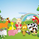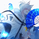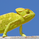1206浏览
Introduction

Inspired from the Kalman filter this indicator aim to provide a good result in term of smoothness and reactivity while letting the user the option to increase/decrease smoothing.

This indicator is constructed in the same manner as many adaptive moving averages by using exponential averaging with a smoothing variable, this is described by :

x= x_1 + a(y - x_1)

where y is the input price (measurements) and a is the smoothing variable, with Kalman filters a is often replaced by K or Kalman Gain, this Gain is what adjust the estimate to the measurements. In the indicator K is calculated as follow :

K = Absolute Error of the estimate/(Absolute Error of the estimate + Measurements Dispersion * length)

The error of the estimate is just the absolute difference between the measurements and the estimate, the dispersion is the measurements standard deviation and length is a parameter controlling smoothness. K adjust to price volatility and try to provide a good estimate no matter the size of length. In order to increase reactivity the price input (measurements) has been summed with the estimate error.

Now this indicator use a fraction of what a Kalman filter use for its entire calculation, therefore the covariance update has been discarded as well as the extrapolation part.

About parameters length control the filter smoothness, the lag reduction option create more reactive results.

Conclusion

You can create smoothing variables for any adaptive indicator by using the : a/(a+b) form since this operation always return values between 0 and 1 as long as a and b are positive. Hope it help !

Patreon: https://www.patreon.com/alexgrover

You can also check out some of the indicators I made for luxalgo : https://www.tradingview.com/u/LuxAlgo/#published-scripts

## 评论Very nice work Alex; appreciated your generosity and efforts as always =DICEKI
@ICEKI, Thanks, i'am on fire, i will try to keep going :)sudhir.mehta
@sudhir.mehta, Will update to version 3, thanks for the comment.Realy great.
Here is the code for version3

dynaf(src,length,lagred)=>
out =0.0
K =na
AC = lagred ? 1 : 0
src_= src + (src - nz(out,src))
out := nz(out,src_) + nz(K)*(src_ - nz(out,src_)) + AC*(nz(K)*(src_ - sma(src_,length)))
K := abs(src_ - out)/(abs(src_ - out) + stdev(src_,length)*length)
out

I use it inside the "Ergotic MDI (Mean Deviation Indicator)" from HPotter
smooth the indicator -> xEMA_S with twoo lines.
1min BTCUSC
length MDI
first line length dynaf 170 smooth 42 with lagred
second line length dynaf 170 smooth 52 with lagred

Really nice to work with your indicators.xcromo
@xcromo, Thanks for the support. Please next time just say you want the script to be in version 3, i would appreciate that.